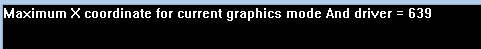Open in App
Not now

# getmaxx() function in C

• Difficulty Level : Easy
• Last Updated : 25 Jan, 2018

The header file graphics.h contains getmaxx() function which returns the maximum X coordinate for current graphics mode and driver.
Syntax :

```int getmaxx();
```

Below is the implementation of getmaxx() function:

 `// C Implementation for getmaxx()``#include ``#include `` ` `// driver code``int` `main()``{``    ``// gm is Graphics mode which is``    ``// a computer display mode that``    ``// generates image using pixels.``    ``// DETECT is a macro defined in``    ``// "graphics.h" header file``    ``int` `gd = DETECT, gm;``    ``char` `arr;`` ` `    ``// initgraph initializes the``    ``// graphics system by loading a``    ``// graphics driver from disk``    ``initgraph(&gd, &gm, ``""``);`` ` `    ``// sprintf stands for “String print”.``    ``// Instead of printing on console, it``    ``// store output on char buffer which``    ``// are specified in sprintf``    ``sprintf``(arr, ``"Maximum X coordinate for current "``        ``"graphics mode And driver = %d"``, getmaxx());`` ` `    ``// outtext function displays text at``    ``// current position.``    ``outtext(arr);`` ` `    ``getch();`` ` `    ``// closegraph function closes the``    ``// graphics mode and deallocates``    ``// all memory allocated by``    ``// graphics system .``    ``closegraph();`` ` `    ``return` `0;``}`

Output :

``````
My Personal Notes arrow_drop_up